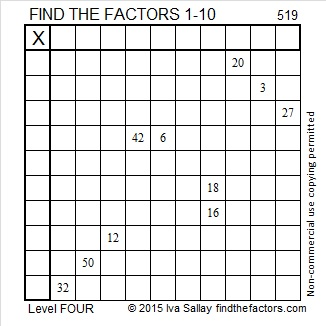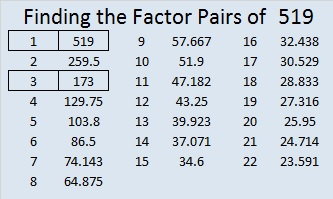# 519 and Level 4

519 = 167 + 173 + 179, three consecutive primes. The difference between each of those prime numbers is 6 so one of them is also one of the factors of 519. The other factor that pairs up with it should be obvious, too.

519 is the hypotenuse of the Pythagorean triple 156-495-519. Can you find the greatest common factor of those three numbers?Print the puzzles or type the solution on this excel file: 10 Factors 2015-06-08

—————————————————————————————————

• 519 is a composite number.
• Prime factorization: 519 = 3 x 173
• The exponents in the prime factorization are 1 and 1. Adding one to each and multiplying we get (1 + 1)(1 + 1) = 2 x 2 = 4. Therefore 519 has exactly 4 factors.
• Factors of 519: 1, 3, 173, 519
• Factor pairs: 519 = 1 x 519 or 3 x 173
• 519 has no square factors that allow its square root to be simplified. √519 ≈ 22.781571—————————————————————————————————1.Joseph Nebus